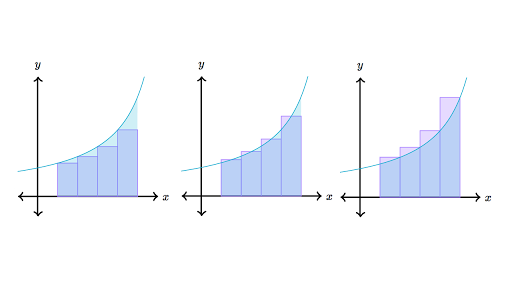# Accumulation & Riemann Sums Assessment Test

10 Questions | Total Attempts: 105SettingsAccumulation and Riemann sums are used when the definite integral represents the area under the curve of a function between two vertical lines but we can't go find this area easily, so we can estimate it using simpler shapes like rectangles.

Related Topics
• 1.
Integrate ∫ 3x+2 dx from x=1 to x=4.
• A.

28.5

• B.

28.6

• C.

28

• D.

28.7

• 2.
Approximate the area under the curve of ∫ cos(x) dx from x=1 to x=5.
• A.

-3.14

• B.

3.14

• C.

-1.8004

• D.

1.8004

• 3.
Integrate f(x)= x^3+4 from x=-2 to x=2 using left riemann sums with four equal subintervals.
• A.

6

• B.

7

• C.

8

• D.

9

• 4.
Integrate and solve ∫ cos(x) dx from x=-11 to x=4.
• A.

3.14

• B.

-1.757

• C.

-3.14

• D.

4.12

• 5.
Integrate and solve ∫ 2x-cosx dx from x=1 to x=6.
• A.

36.121

• B.

24.75

• C.

-36.121

• D.

-24.75

• 6.
Integrate and solve ∫ cos x dx from x=π to x=3π.
• A.

0

• B.

1

• C.

2

• D.

3

• 7.
Integrate and solve ∫1/x +2 dx from x=2 to x=5.
• A.

1n (5/2) +6

• B.

-1n (5/2) +6

• C.

1n (3) +2

• D.

-1n (3) +2

• 8.
Integrate and solve ∫-sin(x)^2-cos(x) from x=12 to x=12.
• A.

-2

• B.

-1

• C.

0

• D.

1

• 9.
Integrate and solve ∫3x^5 dx from x=-5 to x=1.
• A.

-42

• B.

42

• C.

7821

• D.

-7821

• 10.
Integrate and solve ∫2x dx from intervals x=1 to x=3.
• A.

-12

• B.

12

• C.

-8

• D.

8